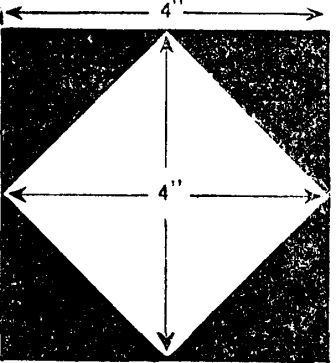John123's Profile
Expert
1299
points

Questions
36

150

John123 loves solving puzzles at PuzzleFry.com. I am proud PuzzleFry member and like my time invested in solving brain teasers.
•Expert Asked on 30th July 2015 in

Arrange the figures in the following way:
and both sums add up alike.
173             85
+ 4            + 92
177            177

• 3741 views
•Expert Asked on 28th July 2015 in

Weary Willie must have worked 1673 days and idled l3Y.! days. Thus the former time, at \$8.00 a day, amounts to exactly the same as the latter at \$10.00

• 5078 views
•Expert Asked on 28th July 2015 in

The amount must have been \$31.63. He received \$63.31. After he had spent a nickel there would remain the sum of \$63.26, which is twice the
amount of the check.

• 3647 views
•Expert Asked on 27th July 2015 in

Make 2 piles with equal number of coins. Now, flip all the coins in one of the pile.

How this will work? lets take an example.

So initially there are 5 heads, so suppose you divide it in 2 piles.

Case:

P1 : H H T T T
P2 : H H H T T

Now when P1 will be flipped
P1 : T T H H H

Another case:

P1 : H T T T T
P2 : H H H H T

Now when P1 will be flipped
P1 : H H H H T

• 7904 views
•Expert Asked on 27th July 2015 in

Now let’s look at some sample paths we can figure out by inspection.

If we start at A and move towards B, we find we can follow the path

RRRUU

(where R = Right one unit, U = Up one unit),

UURRR,
RURUR,
RRUUR,

and so on.

By analyzing our good routes, we see that every good route consists of 5 moves and we have 3 R moves and 2 U moves. We canuse this to generalize a formula to find the number of possible routes.

Since as we’ve shown, order does not matter in our paths (we can have an R in any place of our 5 moves), we can use our combination formula:

C(N,R) = N!/(N-R)! * R!

The number of how many good routes we have can be found by finding how many combinations of 3 R’s we can have in our 5 moves, so we want to calculate:

C(5,3) = 5!/(5-3)! * 2! = 10

• 4770 views
•Expert Asked on 26th July 2015 in

If X is the temperature9X/5 = X — 32
Therefore X=-40C = -40 F

• 4879 views
•Expert Asked on 26th July 2015 in

The entire mile was run in nine minutes. Though fr.>m the facts given we cannot determine the time taken over the first and second quarter-miles separately, we know, however, that together they took four and a half minutes. And the last two quarters were run in two and a quarter minutes each.

• 4116 views
•Expert Asked on 26th July 2015 inThough it does not leave the clear area 4′ x 4′, however does measure 4′ from top to bottom and from side to side.

• 3669 views
•Expert Asked on 26th July 2015 in

We know that there were five droves with an equal number in each drove, and therefore the number must be divisible by 5. As every one of the eight dealers bought the same number of animals, the number must also be divisible by 8. This leads us to the conclusion that the number must be a multiple of 40.
Now the highest possible multiple of 40 that will work will be found to be 120, and this number could be made up in one of two ways—1 cow, 23 sheep and 96 pigs or 3 cows, 8 sheep and 109 pigs. But the first does not fit in because the animals consisted of ‘Cows, Sheep and Pigs’ and a single ‘Cow’ is not ‘Cows’. Therefore the second possibility is the correct answer.

• 5768 views
•Expert Asked on 26th July 2015 in